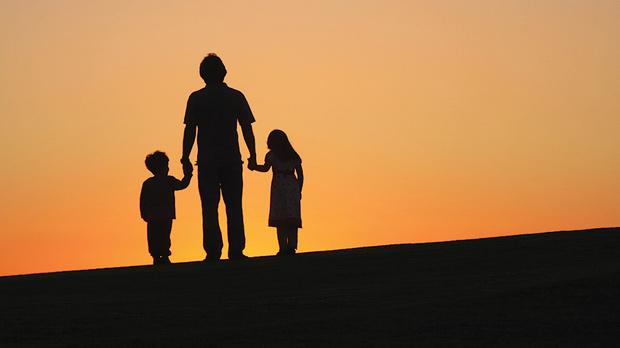# Father age riddle

901.7K Views

Jacob have three children.

The youngest is the same age as the first digit in Jacob age, another is the same age as the second digit in Jacob age, and the third is the same age as the sum of the two digits in Jacob age.

The total of their ages is 33.

How old is Jacob ?Jacob is 23, the children are aged 2, 3 and 5.

Deduction:

Let the ages of Jacob and children be A, B, C and D. Also, let the first and second digits of Jacob’s age be X and Y.
So, we have:
A = 10X + Y  (1)
B = X             (2)
C = Y             (3)
D = X + Y      (4)
Since the sum of the ages is 33, we have
A + B + C + D = 33 => (10X + Y) + X + Y + (X + Y) = 33
=> 12X + 3Y = 33   (5)
Dividing by 3, we get
4X + Y = 11     (6)

The above equation states 11 as the sum of a multiple of 4 and another number.
The only multiples of 4 that are less than 11 are 4 and 8.

i.e, 4X = 4 OR 4X = 8 => X = 1 OR X = 2.

X= 1 is impossible because, in that case, to satisfy (6), Y must be 7, which would mean that a 17-yr old Jacob has an 8 Yr-old child, which is impossible.

Hence: X = 2, Y = 3.